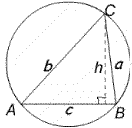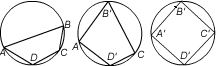#### You may also like### Fixing It

A and B are two fixed points on a circle and RS is a variable diamater. What is the locus of the intersection P of AR and BS?### OK! Now Prove It

Make a conjecture about the sum of the squares of the odd positive integers. Can you prove it?### Summats Clear

Find the sum, f(n), of the first n terms of the sequence: 0, 1, 1, 2, 2, 3, 3........p, p, p +1, p + 1,..... Prove that f(a + b) - f(a - b) = ab.

# Cyclic Triangles

##### Age 16 to 18Challenge Level

Congratulations to Curt, Reigate College and to Andrei, Tudor Vianu National College, Bucharest, Romania for you solutions to this problem

When AB is a diameter angle $ACB$ is 90 degrees so we can use Pythagoras' Theorem. The area of the triangle is given by $\textstyle{1\over 2}ab = \textstyle {1\over 2}ch$ where $h$ is the length of the perpendicular from $C$ to $AB$. Then

$$(a+b)^2=a^2+b^2+2ab=c^2+2ch.$$

Thus $(a+b)^2$ is a maximum when $h$ is a maximum and equal to the radius of the circle $\textstyle {1\over 2}c$ . So the maximum value of $a+b$ is $c\sqrt 2$.When $AB$ is not a diameter we have (using the Cosine Rule):

\eqalign{ (a+b)^2 &= a^2 +b^2 + 2ab \cr &= c^2 + 2ab\cos \angle ACB +2ab \cr &= c^2 +2ab(1+\cos \angle ACB) \cr &=c^2 +4ab \cos^2 \textstyle {1\over 2}\angle ACB.}.

As the area of triangle $ACB$ is given by $\Delta = \textstyle {1\over 2} ab\sin \angle ACB$ we have

\eqalign { (a+b)^2 &= c^2 + {8\Delta \cos^2 \textstyle{1\over 2}\angle ACB \over \sin\angle ACB} \cr &= c^2 + 4\Delta \cot \textstyle {1\over 2}\angle ACB }.

If we keep $A$ and $B$ fixed and vary $C$ then, as $c$ and $\angle ACB$ are constant, and the area of the triangle $\Delta$ is a maximum when $h$ is a maximum, it follows that $a + b$ is a maximum when $h$ is a maximum, that is when $a=b$ and the altitude of the triangle drawn from $C$ to $AB$ is a line of symmetry of the triangle. In this case

$$a=b={c\over 2\sin \textstyle {1\over 2}\angle ACB}.$$

Conjecture: Let $Q$ be a variable cyclic quadrilateral in a circle of radius $r$. Then the area and the perimeter of $Q$ will be a maximum when $Q$ is a square; that is when each of the diagonals of the quadrilateral is a diameter and each diagonal bisects of the other diagonal at right angles.Labelling $Q$ as $ABCD$, consider triangle $ABC$ with $AC$ fixed and $B$ varying. Let $B'$ be the position of $B$ when, by the previous result $AB + BC$ is a maximum, that is when $AB = BC$ Note that this also gives the maximum area of triangle $ABC$. Similarly by considering triangle $ADC$ with $AC$ fixed, we find $D'$ where $AD+DC$ is a maximum and $AD=DC$. Now $B'D'$ is a diameter, keep this fixed and consider triangles $B'CD'$ and $B'AD'$. The positions of $C$ and $A$ that maximise the perimeter and area are $C'$ and $A'$ where $A'C'$ is a diameter. Hence, for the perimeter and area of $Q$ to be a maximum all the sides of the quadrilateral must be equal making $Q$ a square. Hence the maximum perimeter is $4r\sqrt 2$ and the maximum area is $2r^2$.